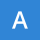Home Communities
IT Knowledge
Inspiration
Languages
EN

JavaScript - how to calculate distance between two points with Pythagorean equation?

17 points
Created by:Aston-Freeman
577

Quick solution:

// P1 = (x1, y1); P2 = (x2, y2)
var a = x2 - x1;
var b = y2 - y1;
var distance = Math.sqrt(a * a + b * b);

Quick example:

// ONLINE-RUNNER:browser;

var a = 3 - 1;
var b = 3 - 1;
var distance = Math.sqrt(a * a + b * b);

console.log(distance); // 2.8284271247461903

1. Overview

Distance calculation between two points is possible with Pythagorean equation. In this article, example how to calculate the distance is presented.

2. Mathematical theorem for coordinate system

Distance calculation for points requires to start with transformation of Pythagorean equation to point version.

a^2 + b^2 = c^2 => c = sqrt(a^2 + b^2)

a = |x2 - x1|

b = |y2 - y1|

c = sqrt(|x2 - x1|^2 + |y2 - y1|^2)

what can be transformed to:

c = sqrt((x2 - x1)^2 + (y2 - y1)^2)

distance beetwen P1 and P2 is equal to c

Note: absolute value of a and b can be avoided because of squares inside Pythagorean equation - squares remove minuses.

Example calculations:

a = |x2 - x1| = |3 - 7| = 4

b = |y2 - y1| = |5 - 2| = 3

c = sqrt(4^2 + 3^2) = sqrt(16 + 9) = sqrt(25) = 5

3. JavaScript custom distance function example

// ONLINE-RUNNER:browser;

function calculateDistance(p1, p2) {
var a = p2.x - p1.x;
var b = p2.y - p1.y;

return Math.sqrt(a * a + b * b);
}

// Example:

var p1 = {x: 7, y: 2};
var p2 = {x: 3, y: 5};

var distance = calculateDistance(p1, p2);

console.log(distance);

4. Math.hypot() method example

Note: Math.hypot() has been introduced in ECMAScript 2015.

// ONLINE-RUNNER:browser;

function calculateDistance(p1, p2) {
var a = p2.x - p1.x;
var b = p2.y - p1.y;

return Math.hypot(a, b);
}

// Example:

var p1 = {x: 7, y: 2};
var p2 = {x: 3, y: 5};

var distance = calculateDistance(p1, p2);

console.log(distance);